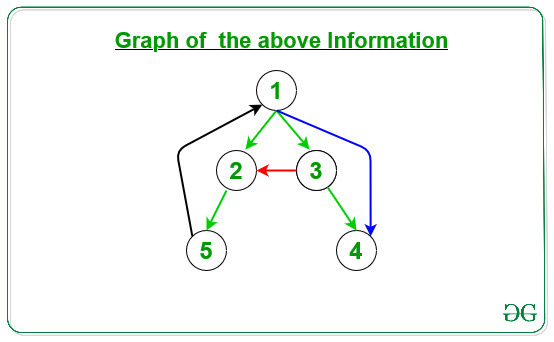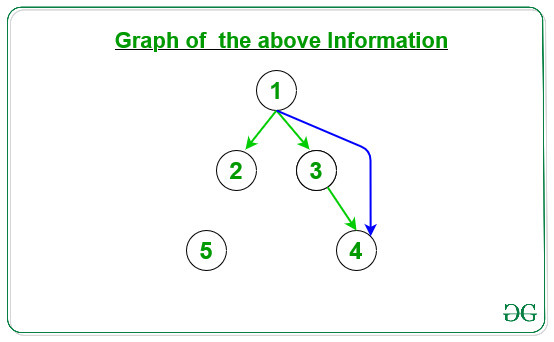# Paranthesis Theorem

Parenthesis Theorem is used in DFS of graph. It states that the descendants in a depth-first-search tree have an interesting property. If v is a descendant of u, then the discovery time of v is later than the discovery time of u.
In any DFS traversal of a graph g = (V, E), for any two vertices u and v exactly one of the following holds:

• The intervals [d[u], f[u]] and [d[v], f[v]] are entirely disjoint and u nor v is a descendant of the other in the depth-first forest.
• The interval [d[u], f[u]] is contained within the interval [d[v], f[v]], and u is a descendant of v in a depth-first tree.
• The interval [d[u], f[u]] is contained entirely within the interval [d[v], f[v]], and v is a descendant of u in a depth-first tree.

Classification of Edges:
DFS traversal can be used to classify the edges of input graph G=(V, E). Four edge types can be defined in terms of a depth-first forest:

1. Tree Edge: It is an edge which is present in the tree obtained after applying DFS on the graph.
2. Forward Edge: It is an edge (u, v) such that v is descendant but not part of the DFS tree.
3. Back edge: It is an edge (u, v) such that v is the ancestor of edge u but not part of the DFS tree. The presence of the back edge indicates a cycle in a directed graph.
4. Cross Edge: It is an edge which connects two-node such that they do not have any ancestor and a descendant relationship between them.

Given a graph of N vertices and M Edges, the task is to classify the M edges into Tree edges, Forward edges, Backward edges and Cross edges.

Examples:

Input: N = 5, M = 7, arr[][] = { { 1, 2 }, { 1, 3 }, { 3, 4 }, { 1, 4 }, { 2, 5 }, { 5, 1 }, { 3, 2 } } }
Output:
{1, 2} -> Tree Edge
{1, 3} -> Tree Edge
{3, 4} -> Tree Edge
{1, 4} -> Forward Edge
{2, 5} -> Tree Edge
{5, 1} -> Backward Edge
{3, 2} -> Cross Edge
Explanation:
1. Green Edges: Tree Edge
2. Blue Edges: Forward Edge
3. Black Edges: Backward Edge
4. Red Edges: Cross Edge
Below is the given graph for the above information:Input: N = 5, M = 4, arr[][] = { { 1, 2 }, { 1, 3 }, { 3, 4 }, { 1, 4 } }
Output:
{1, 2} -> Tree Edge
{1, 3} -> Tree Edge
{3, 4} -> Tree Edge
{1, 4} -> Forward Edge
Explanation:
1. Green Edges: Tree Edge
2. Blue Edges: Forward Edge
3. Black Edges: Backward Edge
4. Red Edges: Cross Edge
Below is the given graph for the above information:Approach:

1. Use DFS Traversal on the given graph to find discovery time and finishing time and parent of each node.
2. By using Parenthesis Theorm classify the given edges on the below conditions:
• Tree Edge: For any Edge (U, V), if node U is the parent of node V, then (U, V) is the Tree Edge of the given graph.
• Forward Edge: For any Edge (U, V), if discovery time and finishing time of node V fully overlaps with discovery time and finishing time of node U, then (U, V) is the Forward Edge of the given graph.
• Backward Edge: For any Edge (U, V), if discovery time and finishing time of node U fully overlaps with discovery time and finishing time of node V, then (U, V) is the Backward Edge of the given graph.
• Cross Edge: For any Edge (U, V), if discovery time and finishing time of node U doesn’t overlaps with discovery time and finishing time of node V, then (U, V) is the Cross Edge of the given graph.

Below is the implementation of the above approach:

 `// C++ program for the above approach ` ` `  `#include "bits/stdc++.h" ` `using` `namespace` `std; ` ` `  `// For recording time ` `int` `tim = 0; ` ` `  `// For creating Graph ` `vector > G; ` ` `  `// For calculating Discovery time ` `// and finishing time of nodes ` `vector<``int``> disc, fin; ` ` `  `// For finding Parent of node ` `vector<``int``> Par; ` ` `  `// For storing color of node ` `vector<``char``> Color; ` ` `  `// Recursive function for DFS ` `// to update the ` `void` `DFS_Visit(``int` `v) ` `{ ` ` `  `    ``// Make the current nodes as visited ` `    ``Color[v] = ``'G'``; ` ` `  `    ``// Increment the time ` `    ``tim = tim + 1; ` ` `  `    ``// Assign the Discovery node of ` `    ``// node v ` `    ``disc[v] = tim; ` ` `  `    ``// Traverse the adjacency list of ` `    ``// vertex v ` `    ``for` `(``auto``& it : G[v]) { ` ` `  `        ``// If the nodes is not visited, ` `        ``// then mark the parent of the ` `        ``// current node and call DFS_Visit ` `        ``// for the current node ` `        ``if` `(Color[it] == ``'W'``) { ` `            ``Par[it] = v; ` `            ``DFS_Visit(it); ` `        ``} ` `    ``} ` `    ``Color[v] = ``'B'``; ` `    ``tim = tim + 1; ` `    ``fin[v] = tim; ` `} ` ` `  `void` `DFS(vector >& G) ` `{ ` ` `  `    ``// Intialise Par, disc, fin and ` `    ``// Color vector to size of graph ` `    ``Par.resize(G.size()); ` `    ``disc.resize(G.size()); ` `    ``fin.resize(G.size()); ` `    ``Color.resize(G.size()); ` ` `  `    ``// Initialise the Par[], Color[], ` `    ``// disc[], fin[] ` `    ``for` `(``int` `i = 1; i < G.size(); i++) { ` `        ``Color[i] = ``'W'``; ` `        ``Par[i] = 0; ` `        ``disc[i] = 0; ` `        ``fin[i] = 0; ` `    ``} ` ` `  `    ``// For every vertex if nodes is ` `    ``// not visited then call DFS_Visit ` `    ``// to update the discovery and ` `    ``// finishing time of the node ` `    ``for` `(``int` `i = 1; i < G.size(); i++) { ` ` `  `        ``// If color is 'W', then ` `        ``// node is not visited ` `        ``if` `(Color[i] == ``'W'``) { ` `            ``DFS_Visit(i); ` `        ``} ` `    ``} ` `} ` ` `  `// Function to check whether ` `// time intervals of x and y overlaps ` `// or not ` `bool` `checkOverlap(``int` `x, ``int` `y) ` `{ ` ` `  `    ``// Find the time intervals ` `    ``int` `x1 = disc[x], y1 = fin[x]; ` `    ``int` `x2 = disc[y], y2 = fin[y]; ` ` `  `    ``// Complete overlaps ` `    ``if` `(x2 > x1 && y1 > y2) { ` `        ``return` `true``; ` `    ``} ` `    ``else` `{ ` `        ``return` `false``; ` `    ``} ` `} ` ` `  `// Function to check which Edges ` `// (x, y) belongs ` `string checkEdge(``int` `x, ``int` `y) ` `{ ` ` `  `    ``// For Tree Edge ` `    ``// If x is parent of y, then it ` `    ``// is Tree Edge ` `    ``if` `(Par[y] == x) { ` `        ``return` `"Tree Edge"``; ` `    ``} ` ` `  `    ``// For Forward Edge ` `    ``else` `if` `(checkOverlap(x, y)) { ` `        ``return` `"Forward Edge"``; ` `    ``} ` ` `  `    ``// For Backward Edge ` `    ``else` `if` `(checkOverlap(y, x)) { ` `        ``return` `"Backward Edge"``; ` `    ``} ` ` `  `    ``else` `{ ` `        ``return` `"Cross Edge"``; ` `    ``} ` `} ` ` `  `// Function call to find the Tree Edge, ` `// Back Edge, Forward Edge, and Cross Edge ` `void` `solve(``int` `arr[], ``int` `N, ``int` `M) ` `{ ` ` `  `    ``// Create graph of N size ` `    ``G.resize(N + 1); ` ` `  `    ``// Traverse each edges ` `    ``for` `(``int` `i = 0; i < M; i++) { ` ` `  `        ``int` `x = arr[i]; ` `        ``int` `y = arr[i]; ` ` `  `        ``// Make Directed graph ` `        ``G[x].push_back(y); ` `    ``} ` ` `  `    ``// DFS call to calculate discovery ` `    ``// and finishing time for each node ` `    ``DFS(G); ` ` `  `    ``// Condition for Tree Edge, Forward ` `    ``// Edges, Backward Edge and Cross Edge ` `    ``for` `(``int` `i = 0; i < M; i++) { ` ` `  `        ``int` `x = arr[i]; ` `        ``int` `y = arr[i]; ` ` `  `        ``// Function call to check Edges ` `        ``cout << ``"{"` `<< x << ``", "` `<< y ` `             ``<< ``"} -> "` `<< checkEdge(x, y) ` `             ``<< endl; ` `    ``} ` `} ` ` `  `// Driver Code ` `int` `main() ` `{ ` ` `  `    ``// Number of Nodes ` `    ``int` `N = 5; ` ` `  `    ``// Number of Edges ` `    ``int` `M = 7; ` ` `  `    ``// Edges for the graph ` `    ``int` `arr[M] ` `        ``= { { 1, 2 }, { 1, 3 }, ` `            ``{ 3, 4 }, { 1, 4 },  ` `            ``{ 2, 5 }, { 5, 1 }, ` `            ``{ 3, 1 } }; ` ` `  `    ``// Function Call ` `    ``solve(arr, N, M); ` ` `  `    ``return` `0; ` `} `

Output:

```{1, 2} -> Tree Edge
{1, 3} -> Tree Edge
{3, 4} -> Tree Edge
{1, 4} -> Forward Edge
{2, 5} -> Tree Edge
{5, 1} -> Backward Edge
{3, 1} -> Backward Edge
```

Attention reader! Don’t stop learning now. Get hold of all the important DSA concepts with the DSA Self Paced Course at a student-friendly price and become industry ready.

My Personal Notes arrow_drop_upCheck out this Author's contributed articles.

If you like GeeksforGeeks and would like to contribute, you can also write an article using contribute.geeksforgeeks.org or mail your article to contribute@geeksforgeeks.org. See your article appearing on the GeeksforGeeks main page and help other Geeks.

Please Improve this article if you find anything incorrect by clicking on the "Improve Article" button below.